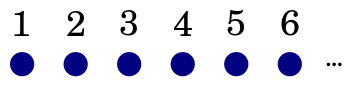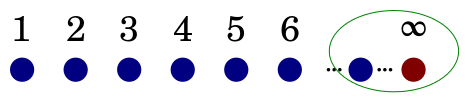Next Topology of Data Types 24

# One-point compactification• Open subsets of ℕ* are:

• Open (i.e., all) subsets of ℕ

• Sc ∪ {∞} where S is a compact (i.e., finite) subset of _ℕ_

• C ∪ {∞} where C is a cofinite subset of _ℕ_• This new space is not discrete

• { ∞ } is not open

• It is still Hausdorff

• { x | x ≠ ∞ } is open